Sequences

Sequences

In this mini-lesson, we will explore the world of sequences in math. You will get to learn about the types of sequences, examples of sequences, formulas for sequences, and other interesting facts around the topic.

You can also check out the playful calculators to know more about the lesson and try your hand at solving a few interesting practice questions at the end of the page.

Let's start by answering a simple question. Have you ever observed patterns in our day-to-day life?

For example, Olivia and Betty are walking along a street to visit their friend, Hailey.

As they walk, they observe a pattern of house numbers on the street.

These numbers are in ascending order.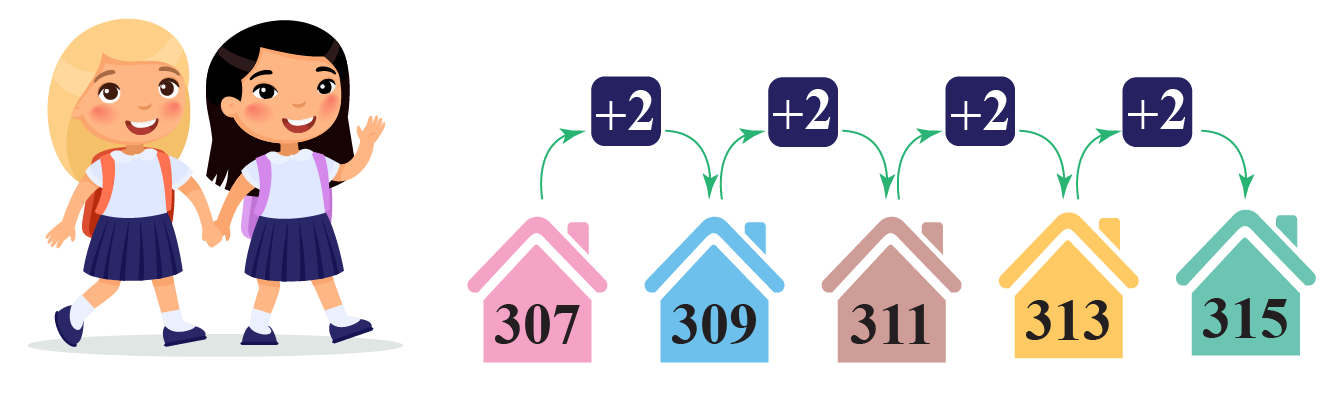Here, these house numbers form a sequence.

Lesson Plan

 1 What Is a Sequence? 2 Important Notes on Sequences 3 Solved Examples on Sequences 4 Thinking out of the Box! 5 Interactive Questions on Sequences

What Is a Sequence?

Definition of Sequence

The sequence is a list of numbers (or items) that exhibits a particular pattern.

Example of Sequence

For example, Olivia has been offered a job with a starting monthly salary of $1000 with an annual increment of$500.Can you calculate her monthly salary in the first three years?

It will be $1000,$1500, and $2000 Observe that Olivia's salary over a number of years forms a sequence. What Is the Order of a Sequence? The order of a sequence can be either in ascending order or in descending order. Ascending Order Look at the sequence below.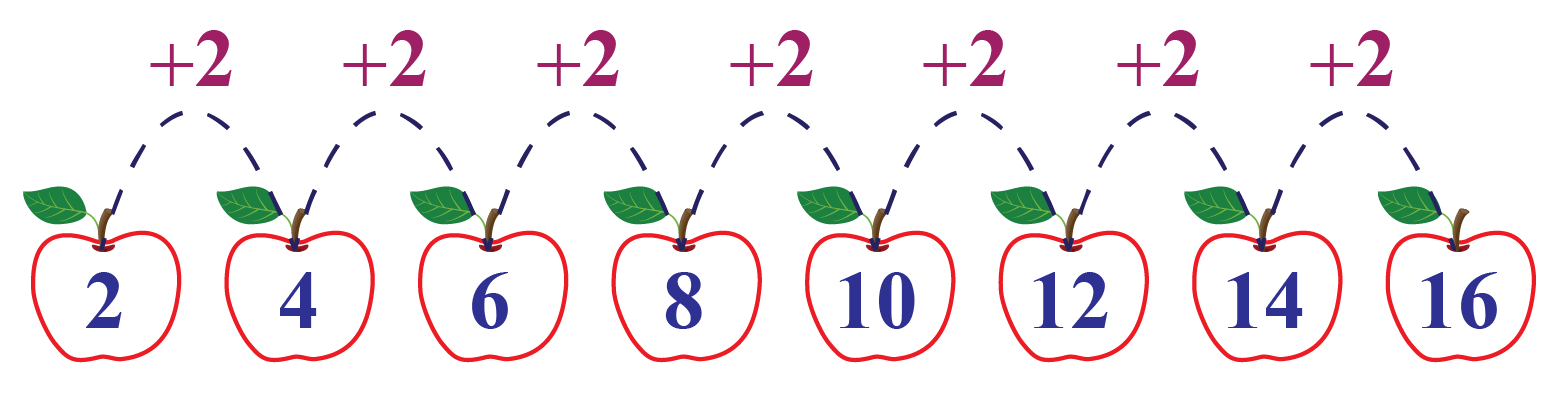Observe that each term is 2 more than the term preceding it. Descending Order In the sequence shown below, each term is 4 less than the term preceding it.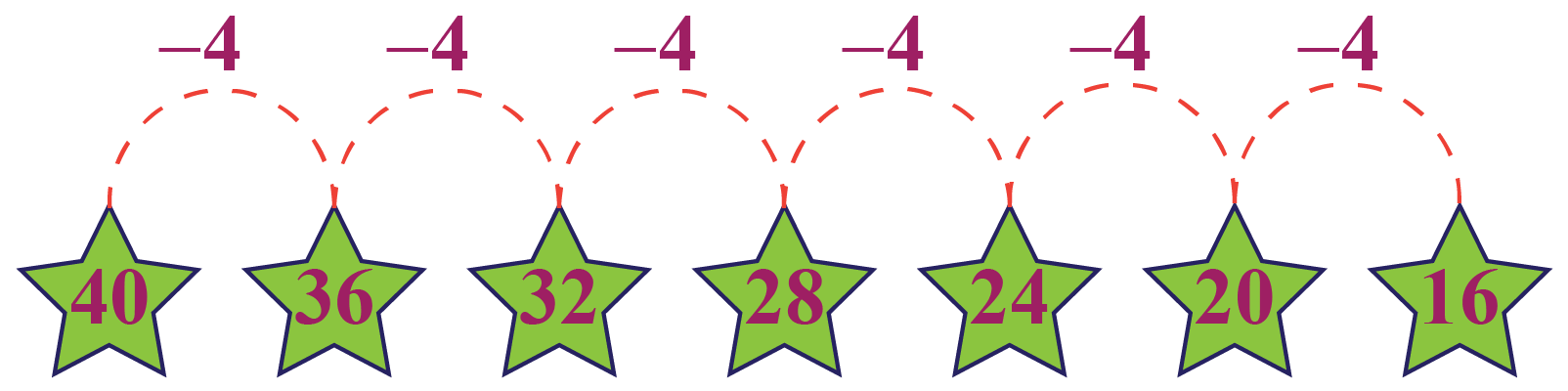Not only this, a sequence can even have an alternate order. Look at the bracelet shown below.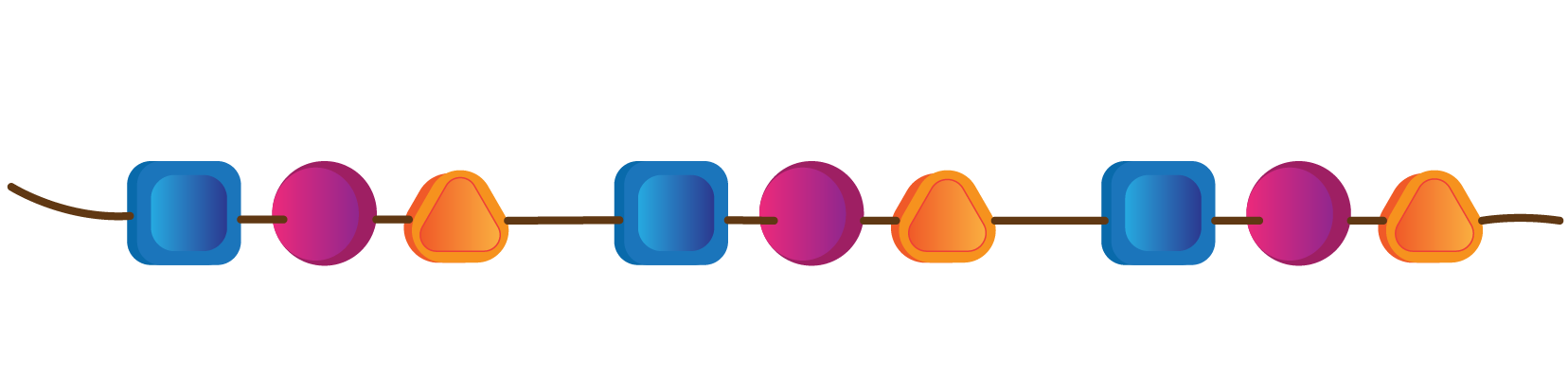Observe that the shapes square, circle, and triangle follow an alternate pattern. What Are the 4 Types of Sequences? The four types of sequences in math are given below. Types of Sequences Examples Arithmetic Sequence 1, 5, 9, 13, ... Geometric Sequence 3, 9, 27, 81, ... Fibonacci Sequence 0, 1, 1, 2, 3, ... Harmonic Sequence $$\dfrac{1}{5}$$, $$\dfrac{1}{10}$$, $$\dfrac{1}{15}$$, ... Infinite or Finite Sequences Finite Sequence A sequence having a finite number of terms is called a finite sequence. Example For example, a sequence of the number of bounces a ball takes to come to the rest. Infinite Sequence A sequence having an infinite number of terms is called an infinite sequence. Example For example, a sequence of natural numbers forms an infinite sequence: 1, 2, 3, 4, and so on. Sequences Like a Set A sequence is like a set. The only difference is that a sequence has a specific pattern that exhibits a rule and the same value can be repeated over time. For example, a sequence containing numbers 0 and 1 can be 1, 1, 0, 0, 1, 1, 0, 0, and so on. But a set is just {0, 1}.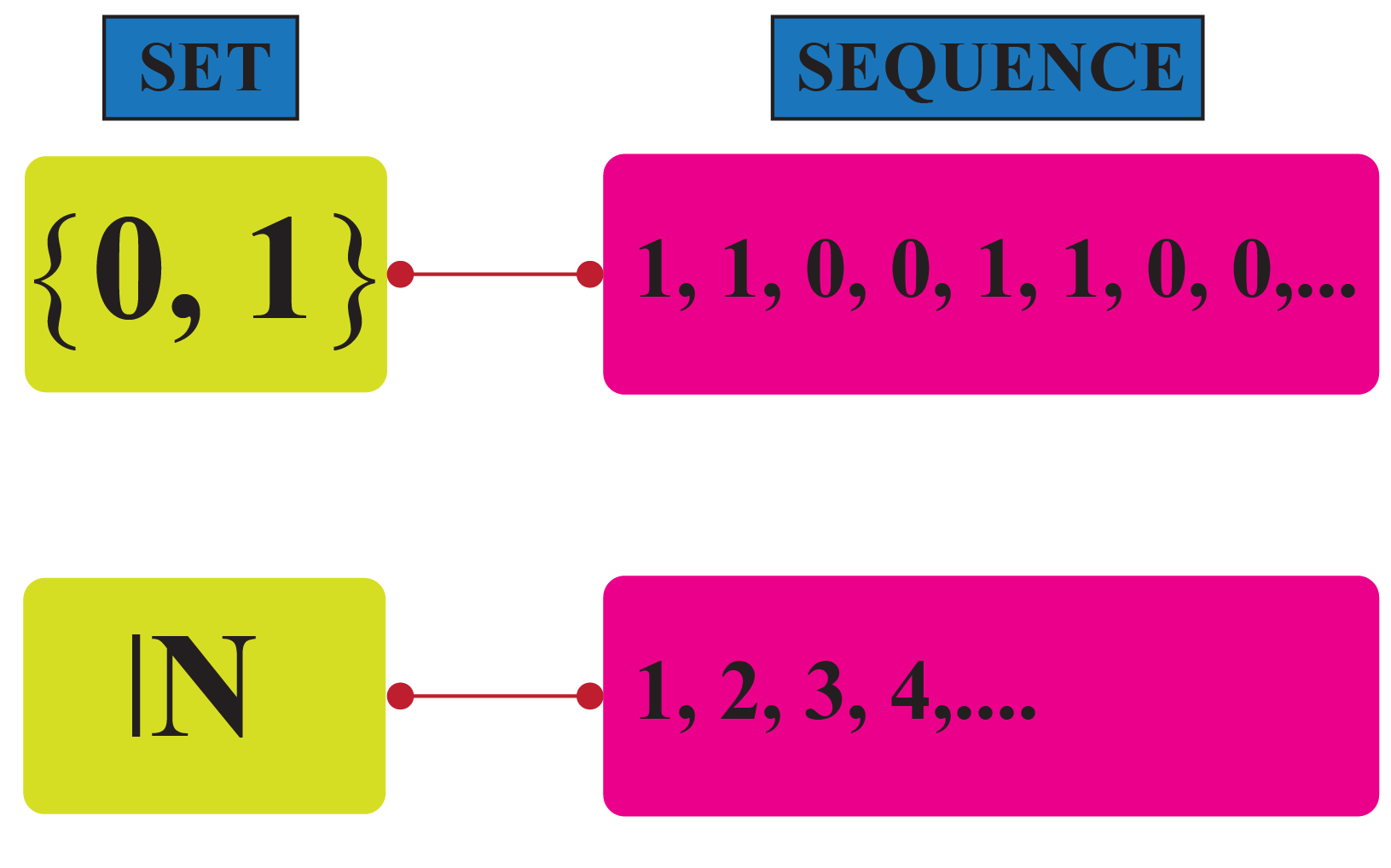Many Rules of Sequences Most of the sequences exhibit a rule. Examples • A sequence of even numbers follows a rule $$2n$$, where $$n \in \mathbb{N}$$. • A sequence of odd numbers follows a rule $$2n+1$$, where $$n \in \mathbb{N}$$. • A sequence of natural numbers follows a rule $$n$$, where $$n \in \mathbb{N}$$.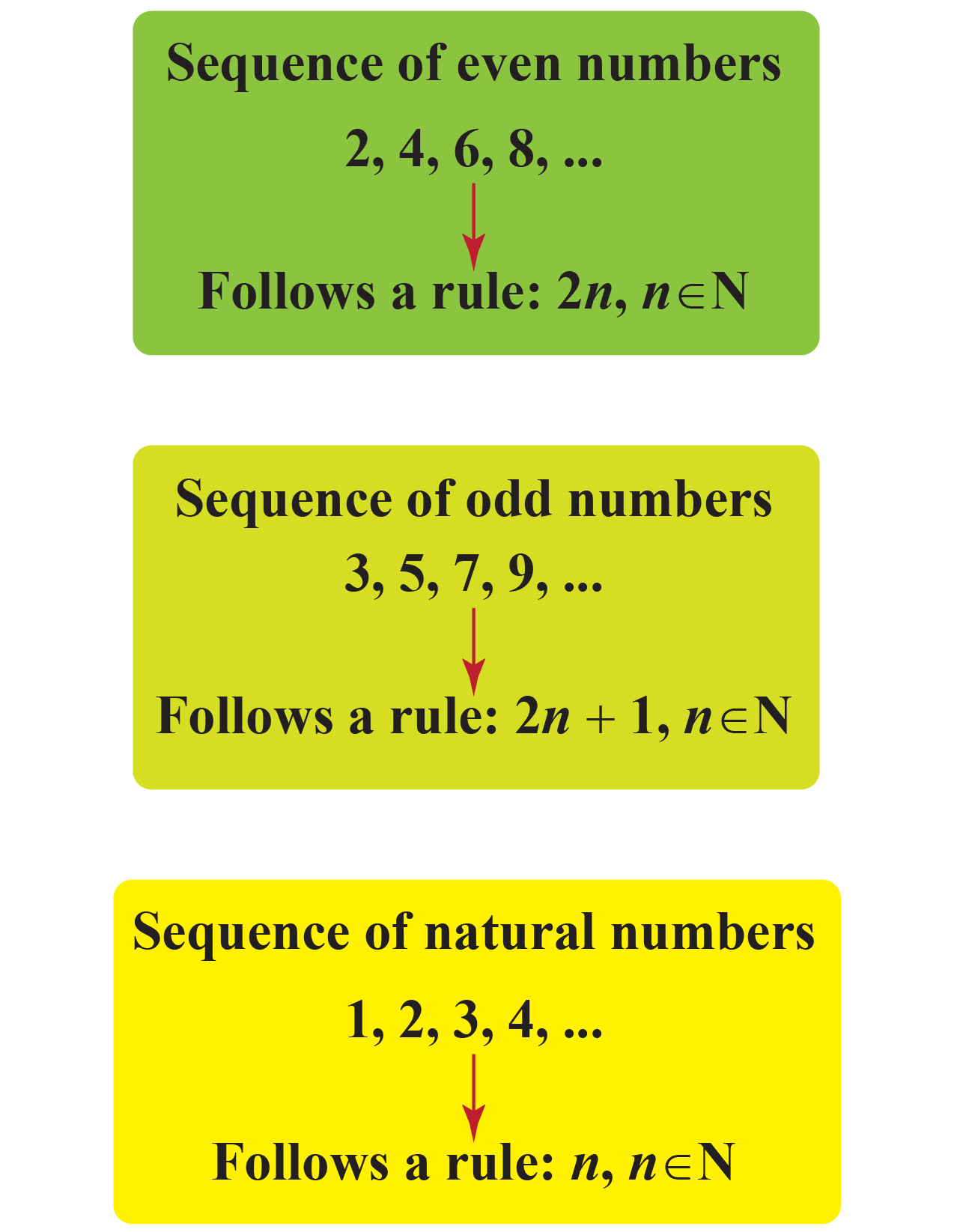Special Sequences in Math There are a few special sequences like arithmetic sequence, geometric sequence, Fibonacci sequence, harmonic sequence, triangular number sequence, square number sequence, and cube number sequence. We will discuss these sequences in detail.Arithmetic Sequence An arithmetic sequence is a sequence of numbers in which each successive term is a sum of its preceding term and a fixed number. This fixed number is called a common difference.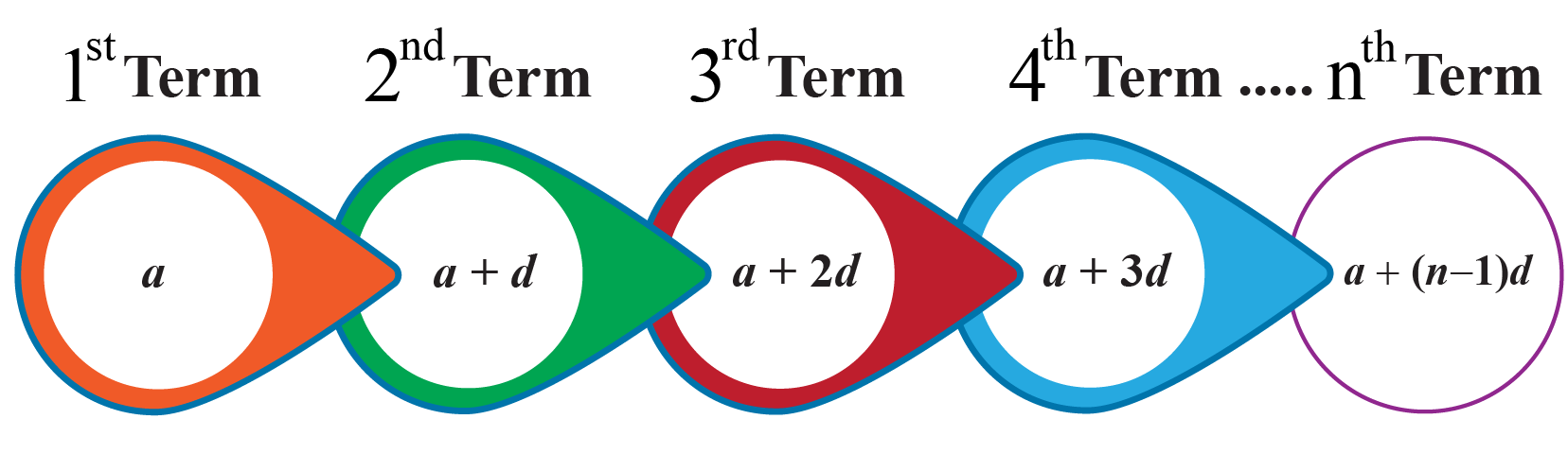Example For example, Mushi put$30 in her piggy bank when she was 7 years old.

She increased the amount on her each successive birthday by $3So, the amount in her piggy bank follows the pattern of$30, $33,$36, and so on.

The succeeding terms are obtained by adding a fixed number, that is, 3 This fixed number is called a common difference. It can be positive, negative, or zero. Geometric Sequence A geometric sequence is a sequence where every term bears a constant ratio to its preceding term.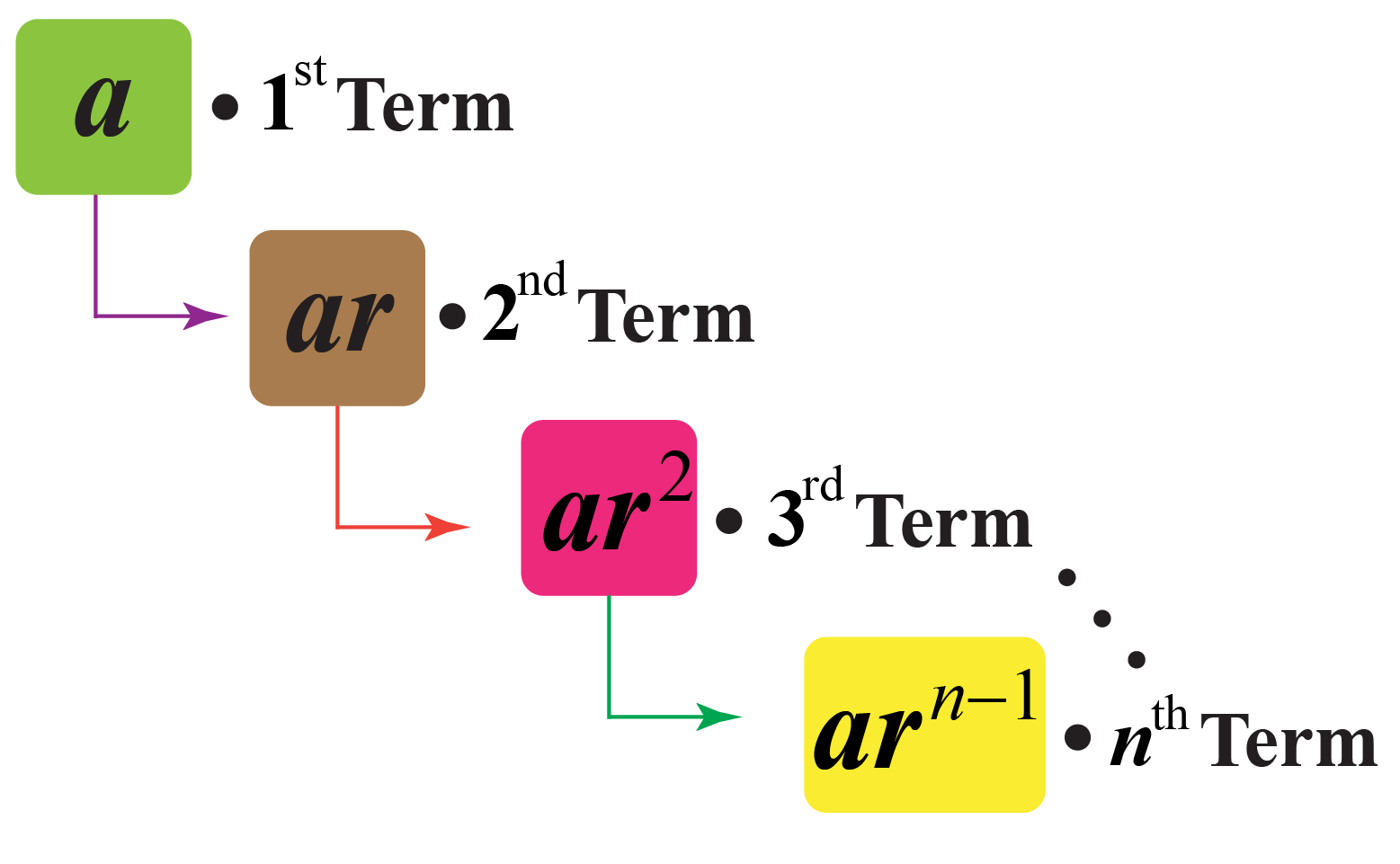Example Consider an example of geometric sequence: 1, 4, 16, 64, ... Observe that $$\dfrac{4}{1}=\dfrac{16}{4}=\dfrac{64}{16}=4$$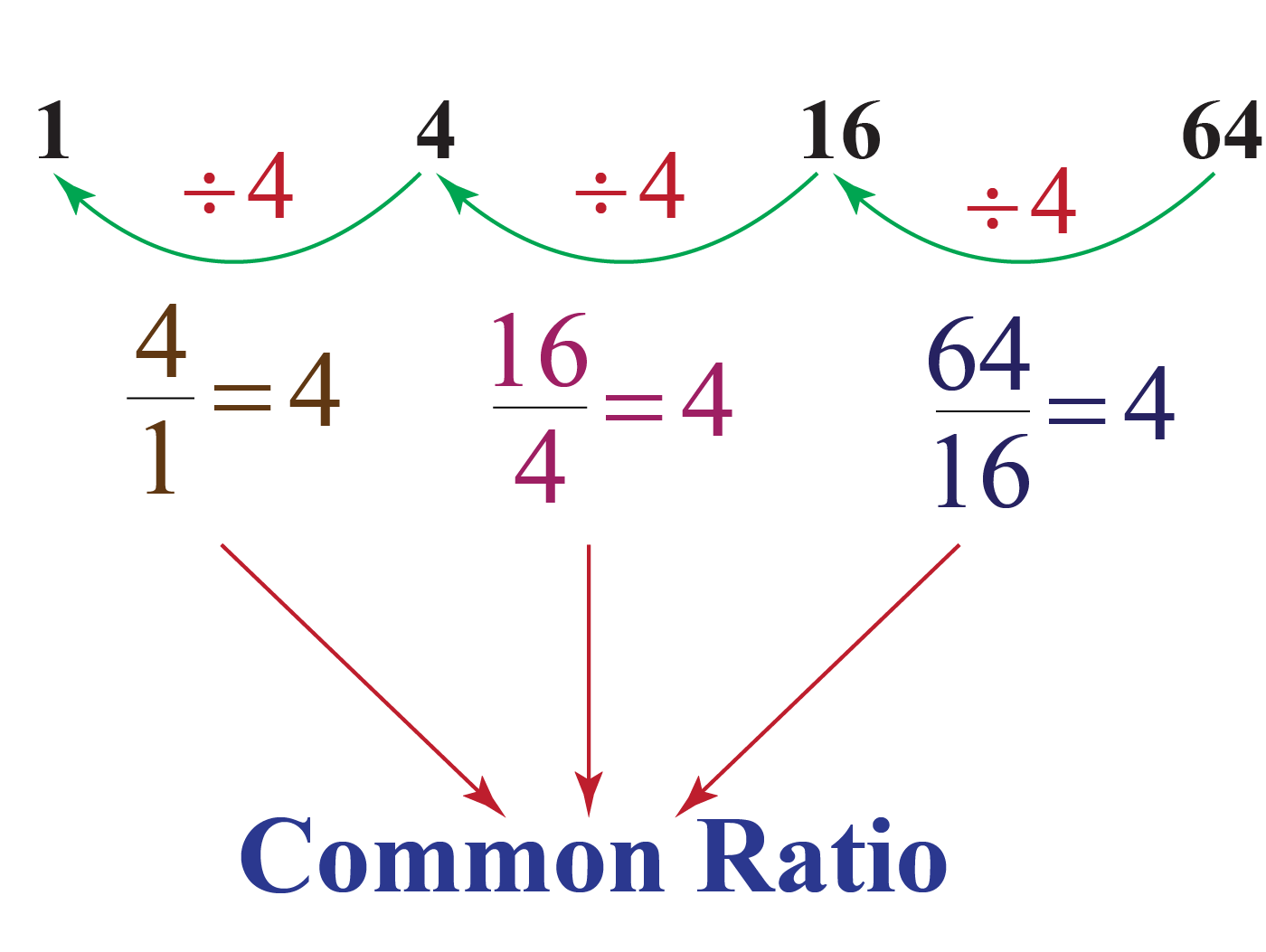Here, 4 is a common ratio. Harmonic Sequence A harmonic sequence is a sequence obtained by taking the reciprocal of the terms of an arithmetic sequence. The sequence of natural numbers is an arithmetic sequence. So, taking reciprocals of each term we get: $1, \dfrac{1}{2}, \dfrac{1}{3}, \dfrac{1}{4}, ...$ This is harmonic sequence.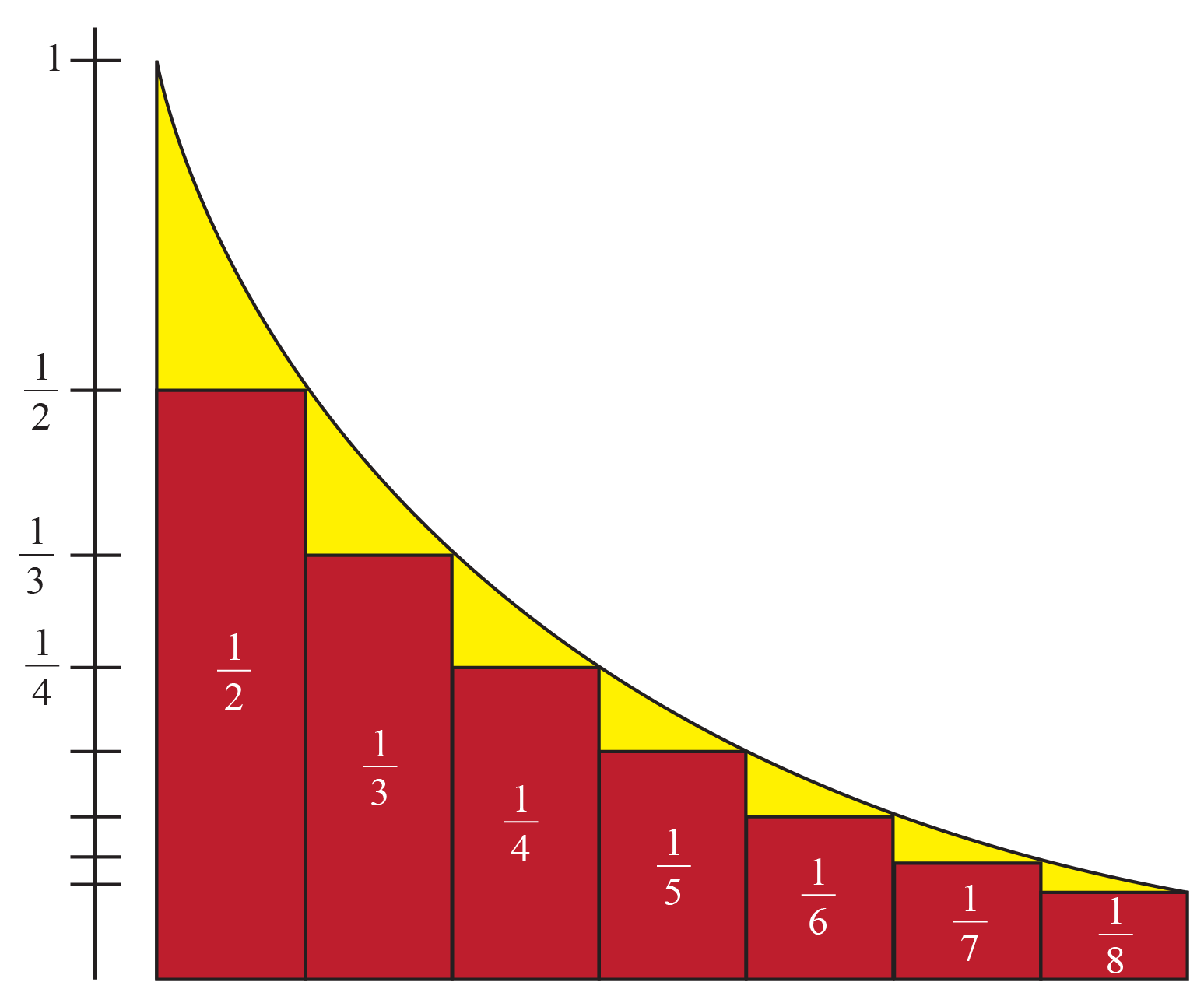Triangular Number Sequence A triangular number sequence is a sequence which is obtained from a pattern forming equilateral triangles. Look at the figure below.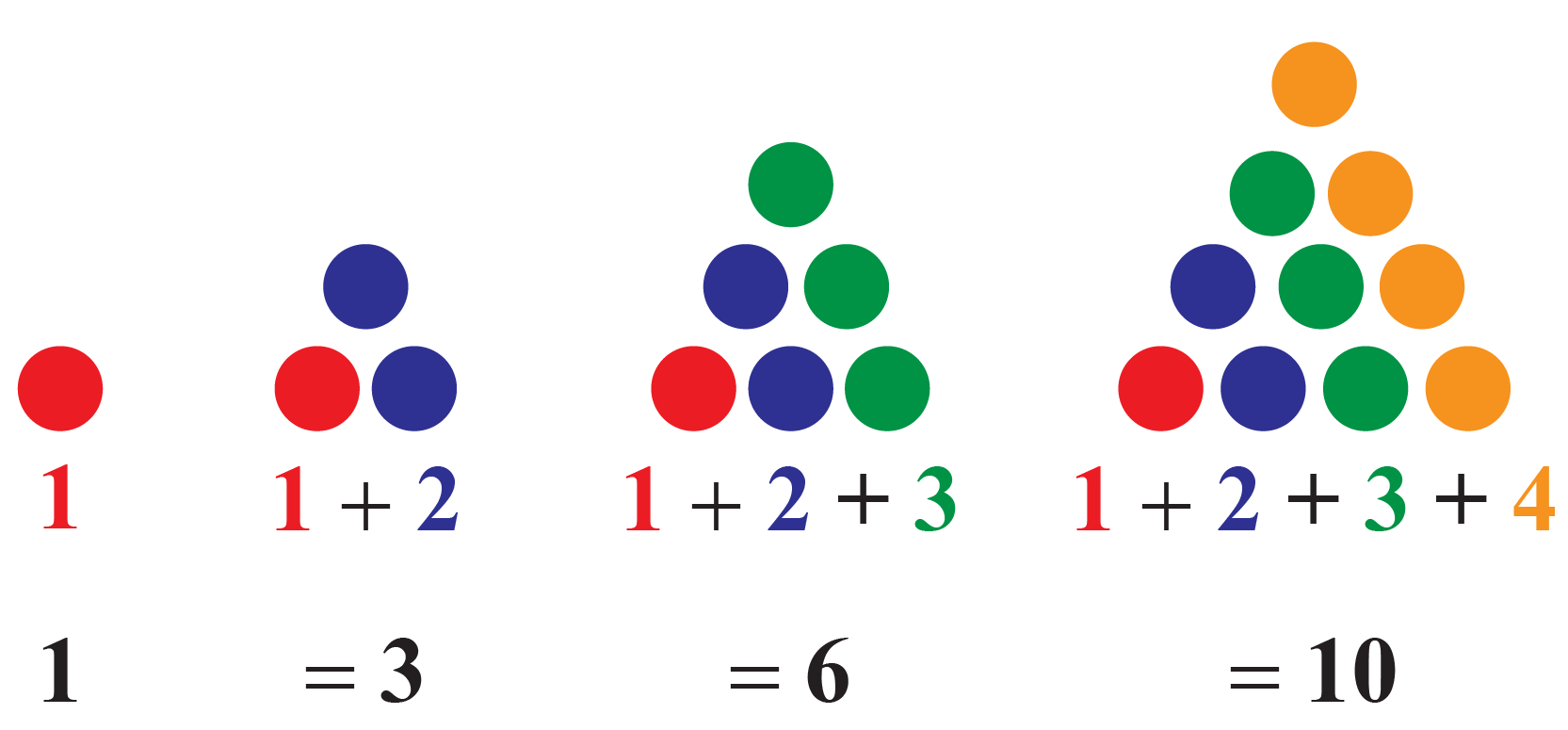The sequence 1, 3, 6, 10, and so on is a triangular number sequence. Square Number Sequence A square number sequence is a sequence which is obtained from a pattern forming squares. Look at the figure below.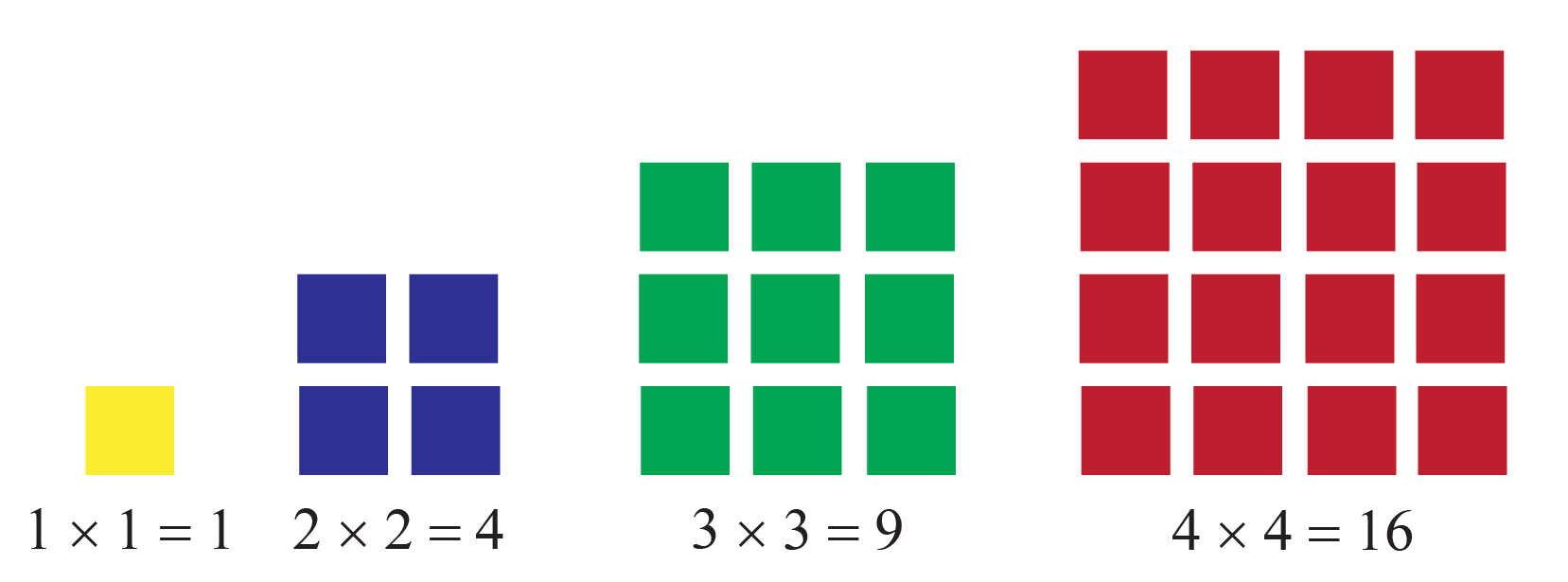The sequence 1, 4, 9, 16, and so on is a square number sequence. Cube Number Sequence A cube number sequence is a sequence which is obtained from a pattern forming cubes. Look at the figure below.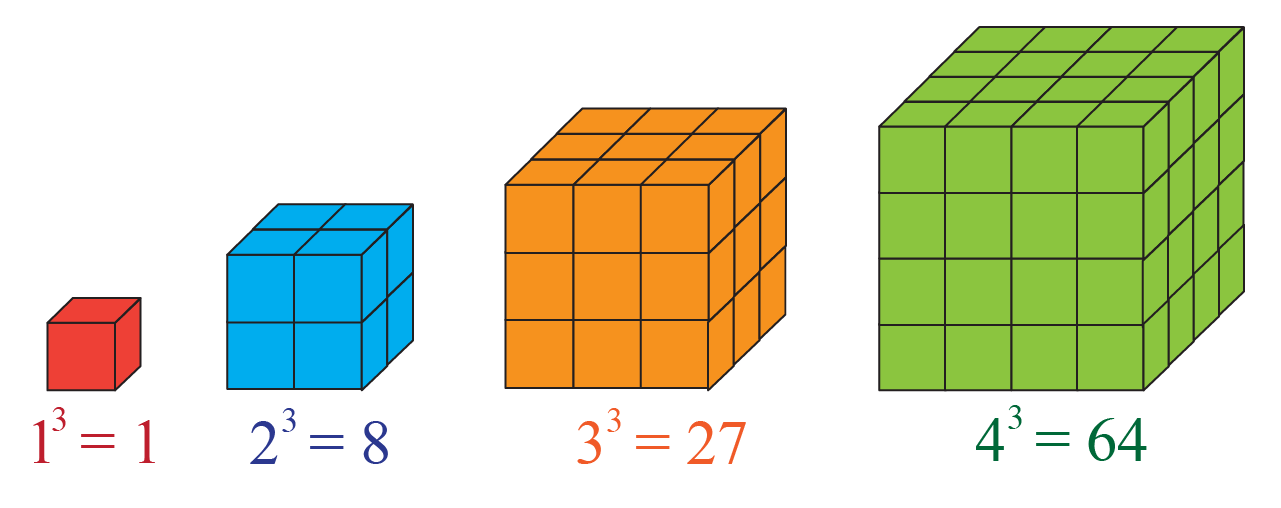The sequence 1, 8, 27, 64, and so on is a cube number sequence. Fibonacci Sequence Fibonacci sequence is a sequence where every term is the sum of the last two preceding terms. Example For example, a pair of rabbits do not reproduce in their 1st month. Starting from the 2nd month and every subsequent month, they reproduce another pair.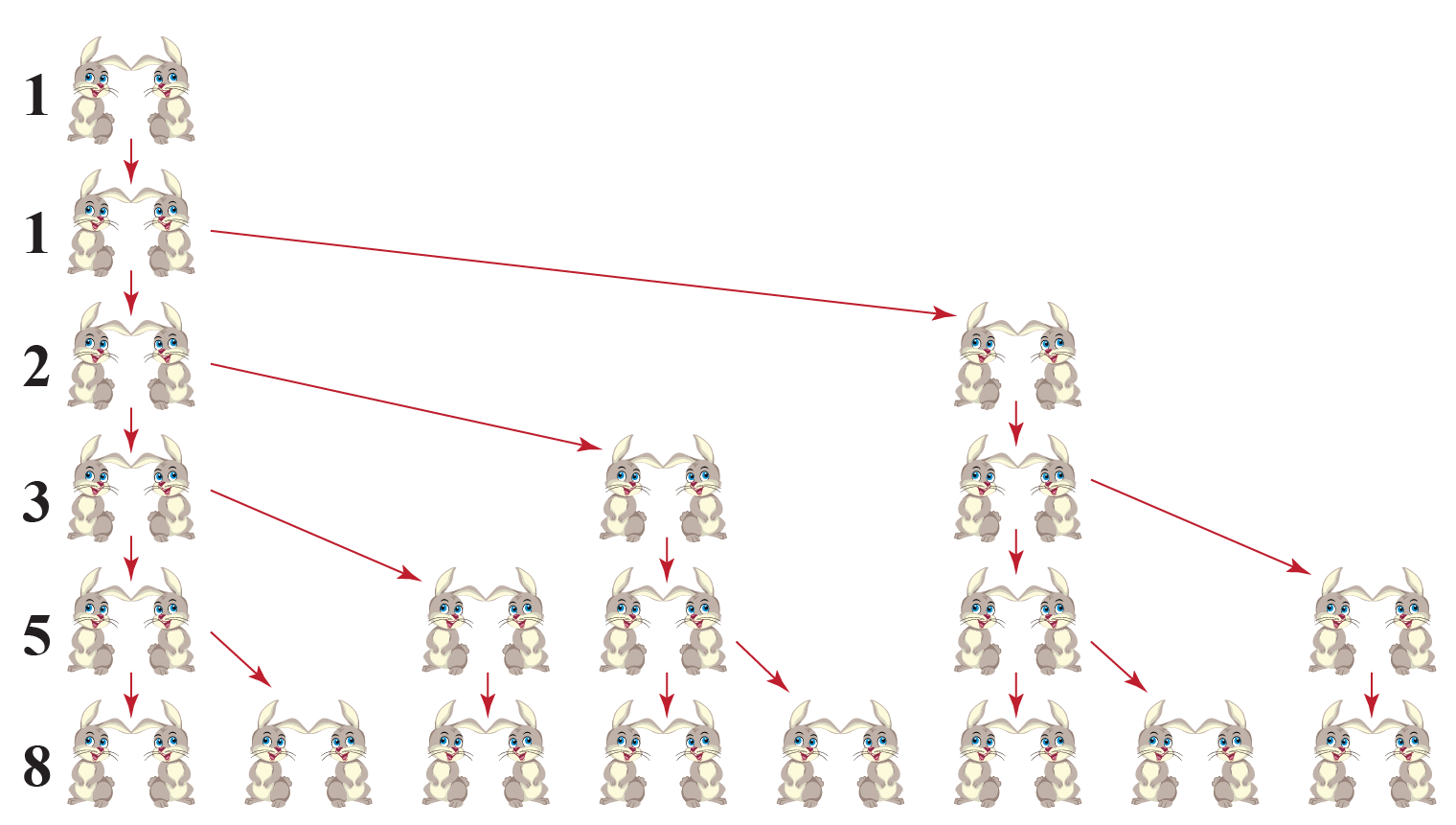So, the sequence of the number of rabbits is 1, 1, 2, 3, 4, 7, 11, and so on. Series and Partial Sums Consider a sequence given by $$a_{1}$$, $$a_{2}$$, $$a_{3}$$, $$a_{4}$$, etc. Then the sum $$a_{1}+a_{2}+a_{3}+a_{4}+...+a_{n}+...$$ is the series associated with the sequence. Series can be represented using sigma notation, $$\sum$$. So, the series is represented as $$\sum_{n=1}^{\infty}a_{n}$$. The partial sum is a part of the series. The sum up to $$k$$ terms in the series $$\sum_{n=1}^{\infty}a_{n}$$ given by $$\sum_{n=1}^{k}a_{n}$$ is partial sum of series. Example Consider a sequence of prime numbers: 2, 3, 5, 7, 11, and so on. The series associated with this is $$\sum_{n=1}^{\infty}a_{n}$$, where $$a_{n}$$ is the $$n^{\text{th}}$$ prime number. Partial sum up to 4 terms is $$2+3+5+7=17$$ Finding a Rule We can have more than one rule for a sequence. For example, the sequence of odd numbers: $$3, 5, 7, 9, ...$$ We can have many rules to define this sequence. • $$2n+1$$, where $$n \in \mathbb{N}$$ • $$a_{1}=3, a_{n+1}=a_{n}+2$$, where $$n \in \mathbb{N}$$ We can infact find more rules defining this sequence. So, now you know the difference between "A Rule" and "The Rule"! What Is the Formula for Sequences? Let's learn the formulas for some special sequences. Arithmetic Sequence Let the first term of the sequence be $$a$$ and the common difference be $$d$$. Then, $$n^{\text{th}}$$ term of the sequence is given by:  \begin{align}a_{n}=a+(n-1)d\end{align} Geometric Sequence Let the first term of the sequence be $$a$$ and the common ratio be $$r$$. Then, $$n^{\text{th}}$$ term of the sequence is given by:  \begin{align}a_{n}=ar^{n-1}\end{align} Harmonic Sequence Let the first term and the common difference of the associated arithmetic sequence be $$a$$ and $$d$$ respectively. Then, $$n^{\text{th}}$$ term of the sequence is given by:  \begin{align}a_{n}=\dfrac{1}{a+(n-1)d}\end{align} Fibonacci Sequence Let first two terms of the sequence be $$a_{1}=0$$ and $$a_{2}=1$$. Then, $$n^{\text{th}}$$ term of the sequence is given by:  \begin{align}a_{n+2}=a_{n}+a_{n+1}\end{align} Square Number Sequence The $$n^{\text{th}}$$ term of the sequence is given by:  \begin{align}a_{n}=n^{2}\end{align} Triangular Number Sequence The $$n^{\text{th}}$$ term of the sequence is given by the sum of first $$n$$ natural numbers.  \begin{align}a_{n}=\sum_{k=1}^{n}k\end{align} Cube Number Sequence The $$n^{\text{th}}$$ term of the sequence is given by:  \begin{align}a_{n}=n^{3}\end{align} Calculator of Sequences Try the simulation below to $$n^{\text{th}}$$ term of these special sequences. Hope this calculator of sequences helped you to practice well. Finding Missing Numbers Use the formulas of special sequences to find the missing terms. Examples For example, consider arithmetic sequence: -32, -29, -26, etc. The 11th term will be given by $$a+10d$$. Here, $$a=-32$$ and $$d=-29+32=3$$. So, $11^{\text{th}}\text{term}=a+10d=-32+10(3)=-2$ Experiment with the simulation below to find the missing terms of different sequences. Finding a Difference In an arithmetic sequence, successive terms are obtained by adding a fixed to its preceding terms. This fixed number is a common difference. Let $$a$$ be the first term and $$d$$ be a common difference. Then, arithmetic sequence is $$a, a+d, a+2d, etc.$$.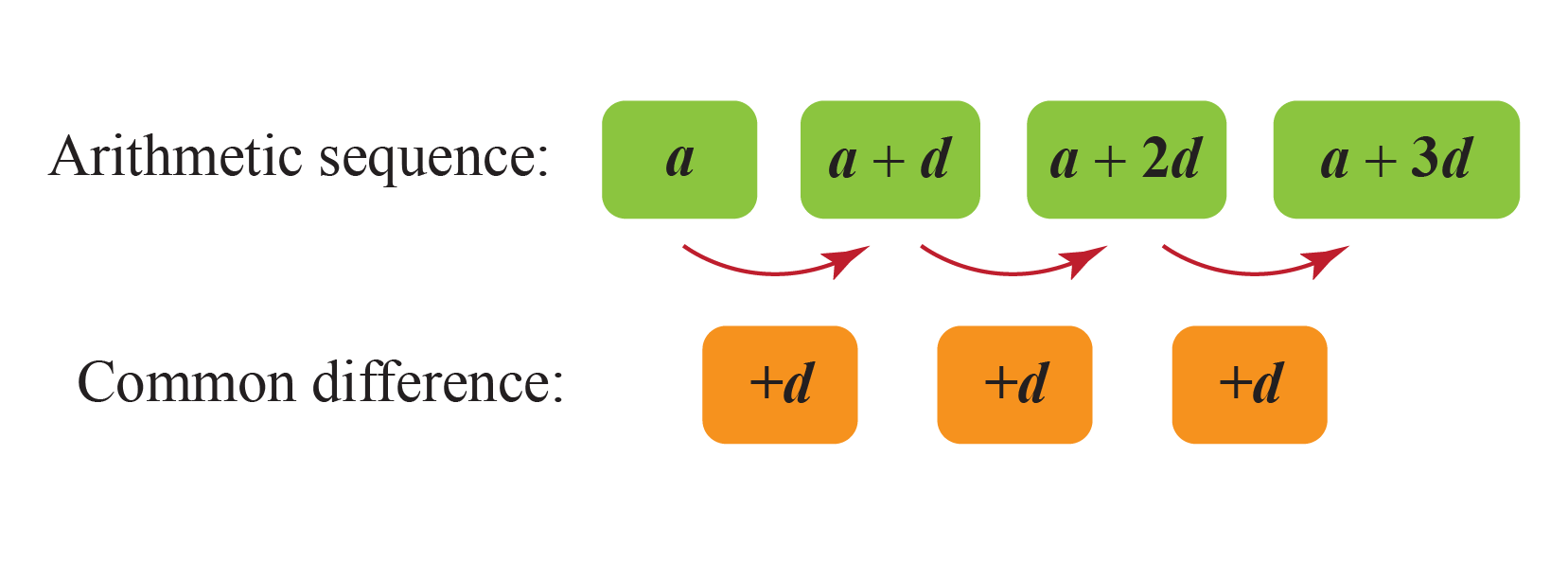Example Consider the sequence: $$2, 5, 8, 11, etc.$$ Common difference is $$5-2=3$$ The number 3 is added every time to obtain the next term. Now, consider the sequence: $$-3, -7, -11, -15, etc.$$ Common difference is $$-7-(-3)=-7+3=-4$$ Second Differences Consider a sequence: $$1, 2, 4, 7, 11, etc.$$ The first difference is the difference between consecutive terms. The second difference is the difference between consecutive first differences.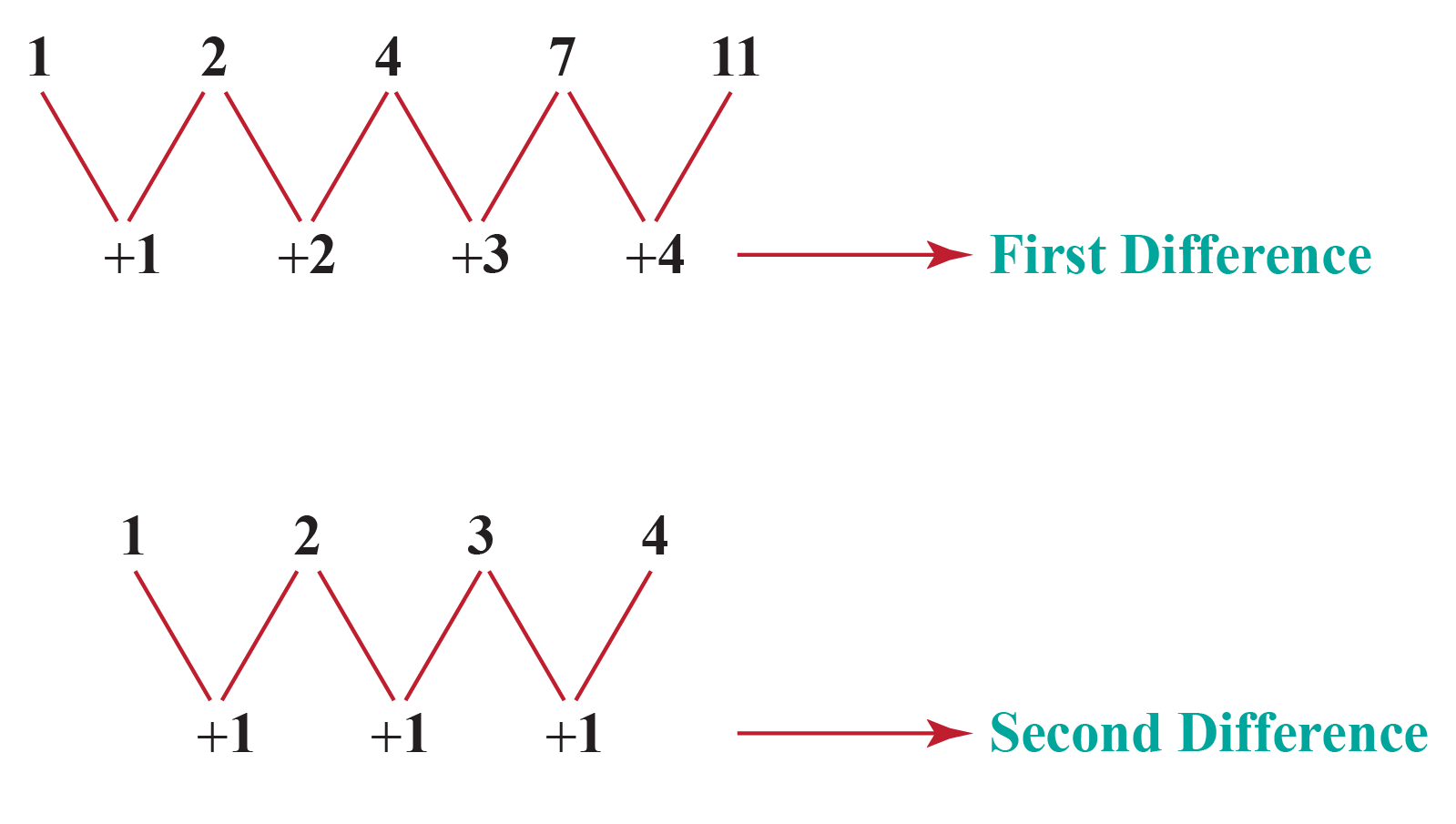Solved Examples on Sequences  Example 1 A taxi charges2 for the first mile and $1.5 for each subsequent mile.How much Katie need to pay to the taxi driver if she travels 5 miles? Solution The taxi fare for the first few miles are$2, $3.5,$5, ...

It forms an arithmetic sequence with a common difference, $$d=1.5$$ and first term, $$a=2$$

The 5th term of the sequence will give the taxi fare for the first 5 miles.

The 5th term of the sequence will be given by $$a+4d$$.

\begin{align}a+4d&=2+4(1.5)\\&=2+6\\&=8\end{align}

 $$\therefore$$ So, Katie pays $$8$$ to the taxi driver for 5 miles.
 Example 2

Flora loves growing flowers in her garden.

There is 1 flower in the 1st row, 4 flowers in the 2nd row, 9 flowers in the 3rd row, and so on.In which row did she plant 100 flowers?

Solution

Observe that the number of flowers follows a square number sequence.

$$n^{\text{th}}$$ term in the square sequence is given by $$n^2$$.

So, in the 10th row, she plants 100 flowers.

 $$\therefore$$ Flora planted 100 flowers in the 10th row.
 Example 3

Look at the pattern shown below.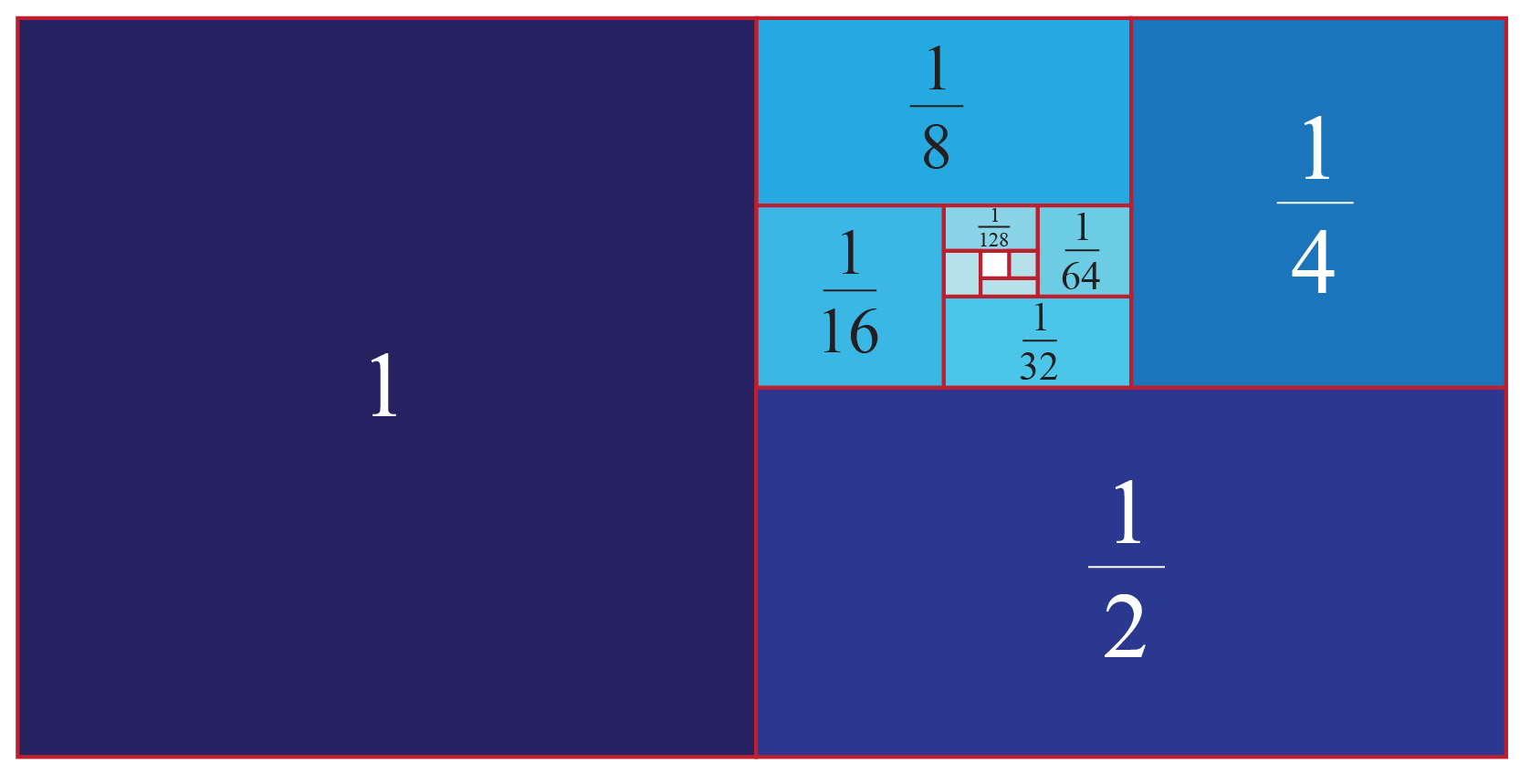Observe that each square is half of the size of the square next to it.

Which sequence does this pattern represent?

Solution

Let's write the sequence represented in the figure.

$1, \dfrac{1}{2}, \dfrac{1}{4}, \dfrac{1}{8}, \dfrac{1}{16},...$

Every successive term is obtained by dividing its preceding term by 2

The sequence exhibits a common ratio of $$\dfrac{1}{2}$$

 $$\therefore$$ The pattern represents the geometric sequence.
 Example 4

Hailey's teacher asks her to fill the missing numbers in the sequences shown on the blackboard.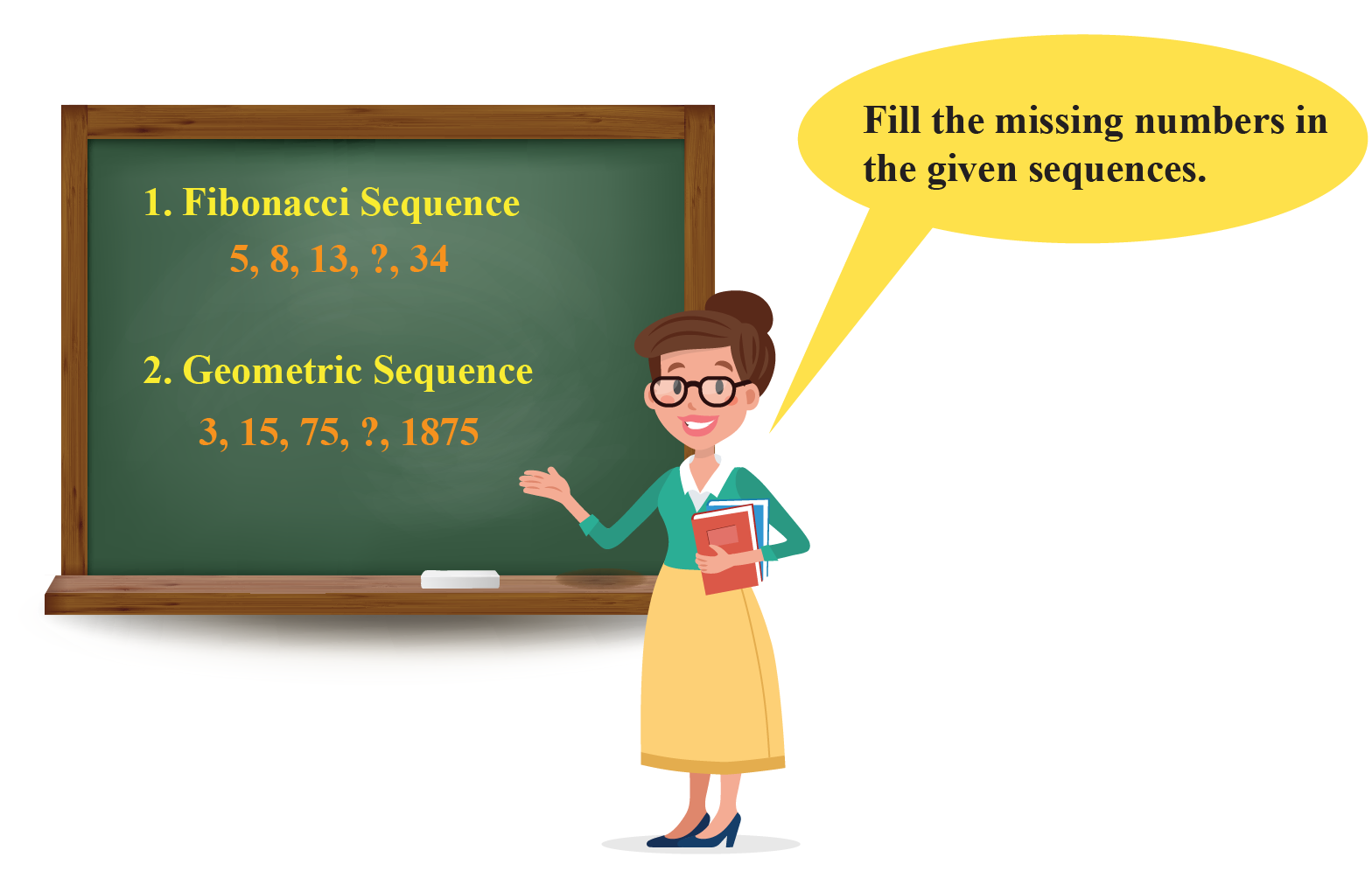Can you help her?

Solution

1. The first sequence is a Fibonacci sequence.

Every term is the sum of the last two terms.

4th term is missing in the sequence.

So, the 4th term is the sum of the 2nd term and 3rd term.

\begin{align}4^{\text{th}}\text{term}&=2^{\text{nd}}\text{term}+3^{\text{rd}}\text{term}\\&=8+13\\&=21\end{align}

2. The second sequence is a geometric sequence.

Every term is the product of the common ratio and the previous term

4th term is missing in the sequence.

The common ratio is $$\dfrac{15}{3}=5$$

So, the 4th term is the product of 5 and the 3rd term.

\begin{align}4^{\text{th}}\text{term}&=\text{Common ratio} \times 3^{\text{rd}}\text{term}\\&=5 \times 75\\&=375\end{align}

 $$\therefore$$ The missing terms are 21 and 375 respectively.
 Example 5

The 6th term and 11th term of the harmonic sequence are 10 and 18 respectively.

Can you find the common difference between the associated arithmetic sequence?

Solution

By definition of the harmonic sequence, we have

6th term of arithmetic sequence = $$\dfrac{1}{10}$$

11th term of arithmetic sequence = $$\dfrac{1}{18}$$

By definition of an arithmetic sequence, we have 2 equations.

\begin{align}a+5d=\dfrac{1}{10}\;\;\;\;\;\; \cdots (1)\end{align}

\begin{align}a+10d=\dfrac{1}{18}\;\;\;\;\;\; \cdots (2)\end{align}

Subtract equation (1) from equation (2)

\begin{align}(a+10d)-(a+5d)&=\dfrac{1}{18}-\dfrac{1}{10}\\5d&=-\dfrac{2}{45}\\d&=-\dfrac{2}{225}\end{align}

 $$\therefore$$ So, the common difference is $$-\dfrac{2}{225}$$Think Tank
 1. The summer Olympics are held after every 4 years.Can you think of any formula to represent the years of the summer Olympics starting from 2012?

Interactive Questions

Here are a few activities for you to practice.

Let's Summarize

We hope you enjoyed learning about sequences with the examples and practice questions. Now, you will be able to easily remember the formulas of sequence and solve problems on sequences in math, which include arithmetic sequence, geometric sequence, harmonic sequence, and other types of sequences.

At Cuemath, our team of math experts is dedicated to making learning fun for our favorite readers, the students!

Through an interactive and engaging learning-teaching-learning approach, the teachers explore all angles of a topic.

Be it worksheets, online classes, doubt sessions, or any other form of relation, it’s the logical thinking and smart learning approach that we, at Cuemath, believe in.

1. What is the process of constructing arithmetic and geometric sequence?

Follow the steps mentioned below to construct an arithmetic sequence.

• Take any number as the first term.
• Fix a number as a common difference.
• Add the common difference to the first term to obtain the second term.
• To obtain each successive term, keep adding the common difference to its preceding term.

Follow the steps mentioned below to construct a geometric sequence.

• Take any number as the first term.
• Fix a number as a common ratio.
• Multiply the common ratio to the first term to obtain the second term.
• To obtain each successive term, keep multiplying the common ratio to its preceding term.
More Important Topics
Numbers
Algebra
Geometry
Measurement
Money
Data
Trigonometry
Calculus
More Important Topics
Numbers
Algebra
Geometry
Measurement
Money
Data
Trigonometry
Calculus